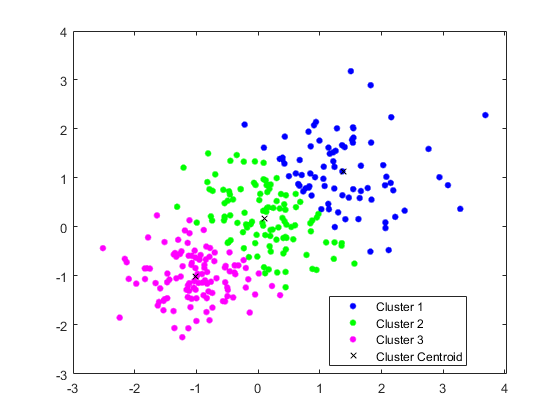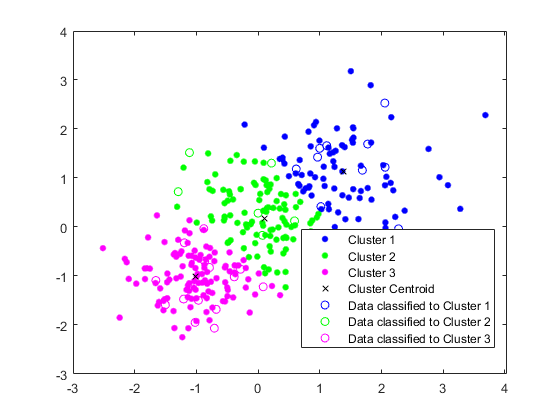• matlab中pdist2函数的具体使用方法： X = rand(3,3) X = 0.5916 0.4424 0.5324 0.3326 0.9044 0.7165 0.8531 0.0332 0.1793 pdist2(X,X) ans = 0 0.5607 0.6004 0.5607 0 1.1482 0.6004 1.1482 0
1、pdist2(X,X)的使用

X=rand(3)

X =

0.8052    0.6568    0.8994
0.3869    0.2429    0.9025
0.3199    0.9852    0.4783

K>> pdist2(X,X)

ans =

0    0.5885    0.7216
0.5885         0    0.8576
0.7216    0.8576         0

怎么计算的呢？将X中的每一行的元素看成一个行矩阵，pdist2(X,X)函数的第一行第一列的元素为X的第一行减去第一行，然后根据欧几里得公式 得 =0

D=X(1,1:3)-X(1,1:3)

D =

0     0     0

K>> pdist2_11=(D*D')^(1/2)

pdist2_11 =

0

pdist2(X,X)函数的第一行第二列的元素为X的第一行减去第二行，然后根据欧几里得公式 得如下

D=X(1,1:3)-X(2,1:3)

D =

0.4183    0.4139   -0.0031
pdist2_12=(D*D')^(1/2)

pdist2_12 =

0.5885

pdist2(X,X)函数的第二行第一列的元素为X的第二行减去第一行，然后根据欧几里得公式 得如下

D=X(2,1:3)-X(1,1:3)

D =

-0.4183   -0.4139    0.0031

K>> pdist2_21=(D*D')^(1/2)

pdist2_21 =

0.5885

其他也是如此！！！

2、pdist2(X,Y)的使用

X = rand(3,3)
Y = [X(:,1:2) rand(3,1)]

X =

0.2874    0.7624    0.6455
0.5017    0.5761    0.1232
0.7615    0.7477    0.5044

Y =

0.2874    0.7624    0.3473
0.5017    0.5761    0.0921
0.7615    0.7477    0.1478

K>> D=pdist2(X,Y)

D =

0.2983    0.6220    0.6875
0.3617    0.0311    0.3124
0.4997    0.5167    0.3565

用同样的方法可得出结果！！！

展开全文• ## matlab中的pdist2函数

万次阅读 2018-09-18 11:31:58
1.pdist2(X) D = pidst(X)主要计算X的行的距离，例如输入X为m*n的矩阵，输出D为m*(m-1)/2的向量，计算方法如下例子： X=[1,2;3,4;5,1] 3*2的矩阵; pdist(X)计算结果为[2.8284,4.1231,3.6056]; 计算方法为第二行...
1.pdist2(X)

D = pidst(X)主要计算X的行的距离，例如输入X为m*n的矩阵，输出D为m*(m-1)/2的向量，计算方法如下例子：

X=[1,2;3,4;5,1] 3*2的矩阵;

pdist(X)计算结果为[2.8284,4.1231,3.6056];

计算方法为第二行与第一行距离(3-1)*(3-1)+(4-2)*(4-2)得到的结果开平方为2.8284，第三行与第一行距离(5-1)*(5-1)+(1-2)*(1-2)得到的结果开平方为4.1231，第三行与第二行距离(5-3)*(5-3)+(1-4)*(1-4)得到的结果开平方为3.6056.

2.pdist(X, Y)

X为a*b矩阵，Y为c*b矩阵，矩阵的每一行代表一个元素，返回一个a*c矩阵，代表X，Y任意两个元素之间的距离。
展开全文• pdist2 两组观测之间的成对距离 句法 D = pdist2(X,Y,Distance) ...D= pdist2(X,Y,Distance)的每一对观测之间的距离。X和Y使用Distance. D= pdist2(X,Y,Distance,DistParameter)指定的度量返回距离。..
pdist2

两组观测之间的成对距离

句法

D = pdist2(X,Y,Distance)

D = pdist2(X,Y,Distance,DistParameter)

D = pdist2(___,Name,Value)

[D,I] = pdist2(___,Name,Value)

描述

D = pdist2(X,Y,Distance)中的每一对观测之间的距离。X和Y使用Distance.

D = pdist2(X,Y,Distance,DistParameter)指定的度量返回距离。Distance和DistParameter。您可以指定DistParameter只有当Distance是'seuclidean', 'minkowski'，或'mahalanobis'.

D = pdist2(___,Name,Value)使用名称-值对参数之一指定附加选项。'Smallest'或'Largest'除了以前语法中的任何参数之外。

例如,

D = pdist2(X,Y,Distance,'Smallest',K)指定的度量计算距离。Distance并返回K与观测的最小成对距离X中的每一次观察Y按升序排列。

D = pdist2(X,Y,Distance,DistParameter,'Largest',K)指定的度量计算距离。Distance和DistParameter并返回K按降序排列的最大成对距离。

[D,I] = pdist2(___,Name,Value)还返回矩阵。I。矩阵I中的观测结果的索引。X对应于D.

实例

计算欧氏距离

用三个观察和两个变量创建两个矩阵。

rng('default') % For reproducibility
X = rand(3,2);
Y = rand(3,2);

计算欧氏距离。输入参数的默认值。Distance是'euclidean'。当不使用名称-值对参数计算欧几里德距离时，不需要指定Distance.

D = pdist2(X,Y)

D = 3×3

0.5387    0.8018    0.1538
0.7100    0.5951    0.3422
0.8805    0.4242    1.2050

D(i,j)对应于观察之间的成对距离i在……里面X和观察j在……里面Y.

计算Minkowski距离

用三个观察和两个变量创建两个矩阵。

rng('default') % For reproducibility
X = rand(3,2);
Y = rand(3,2);

用默认指数2计算Minkowski距离。

D1 = pdist2(X,Y,'minkowski')

D1 = 3×3

0.5387    0.8018    0.1538
0.7100    0.5951    0.3422
0.8805    0.4242    1.2050

计算Minkowski距离，其指数为1，等于城市块距离。

D2 = pdist2(X,Y,'minkowski',1)

D2 = 3×3

0.5877    1.0236    0.2000
0.9598    0.8337    0.3899
1.0189    0.4800    1.7036

D3 = pdist2(X,Y,'cityblock')

D3 = 3×3

0.5877    1.0236    0.2000
0.9598    0.8337    0.3899
1.0189    0.4800    1.7036

找到两个最小的成对距离

用三个观察和两个变量创建两个矩阵。

rng('default') % For reproducibility
X = rand(3,2);
Y = rand(3,2);

找到两个最小的两两欧几里得距离X中的每一次观察Y.

[D,I] = pdist2(X,Y,'euclidean','Smallest',2)

D = 2×3

0.5387    0.4242    0.1538
0.7100    0.5951    0.3422

I = 2×3

1     3     1
2     2     2

中的每一次观察Y, pdist2通过计算并将距离值与X。的每个列中的距离排序。D按升序排列。I中的观测结果的索引。X对应于D.

使用自定义距离函数计算缺少元素的成对距离

定义一个自定义距离函数，该函数忽略NaN值，并使用自定义距离函数计算成对的距离。

用三个观察和三个变量创建两个矩阵。

rng('default') % For reproducibility
X = rand(3,3)
Y = [X(:,1:2) rand(3,1)]

X =

0.8147    0.9134    0.2785
0.9058    0.6324    0.5469
0.1270    0.0975    0.9575

Y =

0.8147    0.9134    0.9649
0.9058    0.6324    0.1576
0.1270    0.0975    0.9706

X和Y的前两列是相同的。假设X(1,1)丢失了。

X(1,1) = NaN

X =

NaN    0.9134    0.2785
0.9058    0.6324    0.5469
0.1270    0.0975    0.9575

计算汉明距离。

D1 = pdist2(X,Y,'hamming')

D1 =

NaN       NaN       NaN
1.0000    0.3333    1.0000
1.0000    1.0000    0.3333

中频观测i在……里面X或观察j在……里面Y含NaN值，函数pdist2回报NaN对于两两之间的距离i和j。因此，D1(1，1)，D1(1，2)和D1(1，3)是NaN价值。

定义自定义距离函数nanhamdist忽略坐标的NaN值并计算Hamming距离。当处理大量的观测时，您可以通过遍历数据的坐标来更快地计算距离。

function D2 = nanhamdist(XI,XJ)
%NANHAMDIST Hamming distance ignoring coordinates with NaNs
[m,p] = size(XJ);
nesum = zeros(m,1);
pstar = zeros(m,1);
for q = 1:p
notnan = ~(isnan(XI(q)) | isnan(XJ(:,q)));
nesum = nesum + ((XI(q) ~= XJ(:,q)) & notnan);
pstar = pstar + notnan;
end
D2 = nesum./pstar;

用nanhamdist的输入参数传递函数句柄。pdist2.

D2 = pdist2(X,Y,@nanhamdist)

D2 =

0.5000    1.0000    1.0000
1.0000    0.3333    1.0000
1.0000    1.0000    0.3333

向现有集群分配新数据并生成C/C++代码

此示例使用：

GPU编码器
MATLAB编码器
统计与机器学习工具箱
执行k-均值聚类

使用三个发行版生成培训数据集。

rng('default') % For reproducibility
X = [randn(100,2)*0.75+ones(100,2);
randn(100,2)*0.5-ones(100,2);
randn(100,2)*0.75];

使用kmeans.

[idx,C] = kmeans(X,3);

绘制星系团和星系团质心图。

figure
gscatter(X(:,1),X(:,2),idx,'bgm')
hold on
plot(C(:,1),C(:,2),'kx')
legend('Cluster 1','Cluster 2','Cluster 3','Cluster Centroid')将新数据分配给现有集群

生成测试数据集。

Xtest = [randn(10,2)*0.75+ones(10,2);
randn(10,2)*0.5-ones(10,2);
randn(10,2)*0.75];

使用现有集群对测试数据集进行分类。从每个测试数据点查找最近的质心，使用pdist2.

[~,idx_test] = pdist2(C,Xtest,'euclidean','Smallest',1);

绘制测试数据并使用idx_test用gscatter.

gscatter(Xtest(:,1),Xtest(:,2),idx_test,'bgm','ooo')
legend('Cluster 1','Cluster 2','Cluster 3','Cluster Centroid', ...
'Data classified to Cluster 1','Data classified to Cluster 2', ...
'Data classified to Cluster 3')输入参数

X,Y — 输入数据
数值矩阵

输入数据，指定为数字矩阵。X是MX-按-n矩阵和Y是我的-按-n矩阵。行对应于单独的观察，列对应于单个变量。

数据类型：single | double

Distance — 距离度量
字符向量 | 串标量 | 功能手柄

距离度量，指定为字符向量、字符串标量或函数句柄，如下表所述。

价值
描述
'euclidean'

欧几里德距离(默认)。

'squaredeuclidean'

平方欧氏距离(本选项仅为提高效率而提供。它不满足三角形不等式。

'seuclidean'

标准化欧氏距离观测值之间的每个坐标差除以标准差的对应元素，S = nanstd(X)。使用DistParameter指定另一个值S.

'mahalanobis'

使用样本协方差的Mahalanobis距离X, C = nancov(X)。使用DistParameter指定另一个值C，其中的矩阵C是对称的正定的。

'cityblock'

城市街区距离。

'minkowski'

明考斯基距离。默认指数为2。DistParameter指定不同的指数P，在哪里P是指数的正标量值。

'chebychev'

切比切夫距离(最大坐标差)

'cosine'

1减去点间夹角的余弦(作为向量处理)。

'correlation'

1减去点之间的样本相关性(作为值的序列处理)。

'hamming'

Hamming距离，即不同坐标的百分比。

'jaccard'

1减去Jaccard系数，这是不同的非零坐标的百分比。

'spearman'

其中一项减去样本Spearman之间的秩相关性(作为值的序列处理)。

@distfun

自定义距离函数句柄。距离函数具有以下形式

function D2 = distfun(ZI,ZJ)
% calculation of distance
...
哪里

ZI是1-按-n包含单一观测的向量。

ZJ是m2-按-n包含多个观察的矩阵。distfun必须接受矩阵ZJ任意数量的观察。

D2是m2-按-1距离向量，以及D2(k)是观察之间的距离ZI和ZJ(k,:).

如果数据不稀疏，通常可以使用内置距离而不是函数句柄来更快地计算距离。

有关定义，请参见距离度量.

当你使用'seuclidean', 'minkowski'，或'mahalanobis'，您可以指定一个附加的输入参数。DistParameter来控制这些指标。您还可以使用这些指标的方式与默认值为DistParameter.

例子： 'minkowski'

DistParameter — 距离度量参数值
正标量 | 数值向量 | 数值矩阵

距离度量参数值，指定为正标量、数字向量或数字矩阵。此参数仅在您指定Distance如'seuclidean', 'minkowski'，或'mahalanobis'.

如果Distance是'seuclidean', DistParameter是每个维度的缩放因子的向量，指定为正向量。默认值是nanstd(X).

如果Distance是'minkowski', DistParameter是Minkowski距离的指数，表示为正标量。默认值为2。

如果Distance是'mahalanobis', DistParameter是一个协方差矩阵，指定为数字矩阵。默认值是nancov(X). DistParameter必须是对称的和正定的。

例子： 'minkowski',3

数据类型：single | double

名称值对参数

指定可选的逗号分隔对Name,Value争论。Name是参数名和Value对应的值。Name必须出现在引号中。可以将多个名称和值对参数按任何顺序指定为Name1,Value1,...,NameN,ValueN.

例子：任一'Smallest',K或'Largest',K。你不能同时使用'Smallest'和'Largest'.

'Smallest' — 要查找的最小距离数
正整数

要查找的最小距离数，指定为由'Smallest'以及一个正整数。如果您指定'Smallest'，然后pdist2的每一列中的距离排序。D按升序排列。

例子：'Smallest',3

数据类型：single | double

'Largest' — 要查找的最大距离数
正整数

要查找的最大距离数，指定为由'Largest'以及一个正整数。如果您指定'Largest'，然后pdist2的每一列中的距离排序。D按降序排列。

例子：'Largest',3

数据类型：single | double

D-成对距离
数值矩阵

成对距离，返回为数字矩阵。

如果您没有指定'Smallest'或'Largest'，然后D是MX-按-我的矩阵，其中MX和我的中的观察数X和Y分别。D(i,j)是观察之间的距离i在……里面X和观察j在……里面Y。中频观测i在……里面X或观察j在……里面Y含NaN，然后D(i,j)是NaN内建距离函数。

如果您指定'Smallest'或'Largest'如K，然后D是K-按-我的矩阵。D包含K最小的或最小的K与观测的最大成对距离X中的每一次观察Y。中的每一次观察Y, pdist2发现K的最小或最大距离，通过计算和比较的距离值与所有的观测X。如果K大于MX, pdist2返回MX-按-我的矩阵。

I-排序索引
正整数矩阵

排序索引，作为正整数矩阵返回。I是和D. I中的观测结果的索引。X对应于D.


展开全文• pdistPairwise distance between pairs of objectsSyntaxD = pdist(X)D = pdist(X,distance)DescriptionD = pdist(X)计算 X 各对行向量的相互距离(X是一个m-by-n的矩阵). 这里 D 要特别注意，D 是一个长为m(m–1)/...
pdistPairwise distance between pairs of objectsSyntaxD = pdist(X)D = pdist(X,distance)DescriptionD = pdist(X)计算 X 中各对行向量的相互距离(X是一个m-by-n的矩阵). 这里 D 要特别注意，D 是一个长为m(m–1)/2的行向量.可以这样理解 D 的生成：首先生成一个 X 的距离方阵，由于该方阵是对称的，且对角线上的元素为0，所以取此方阵的下三角元素，按照Matlab中矩阵的按列存储原则，此下三角各元素的索引排列即为(2,1), (3,1), ..., (m,1), (3,2), ..., (m,2), ..., (m,m–1).可以用命令 squareform(D) 将此行向量转换为原距离方阵.(squareform函数是专门干这事的，其逆变换是也是squareform。)D = pdist(X,distance) 使用指定的距离.distance可以取下面圆括号中的值，用红色标出！MetricsGiven an m-by-n data matrix X, which is treated as m (1-by-n) row vectors x1, x2, ..., xm, the various distances between the vector xs and xt are defined as follows:欧几里德距离Euclidean distance('euclidean')d 2 s,t =(x s x t )(x s x t ) ′  Notice that the Euclidean distance is a special case of the Minkowski metric, where p = 2.欧氏距离虽然很有用，但也有明显的缺点。一：它将样品的不同属性（即各指标或各变量）之间的差别等同看待，这一点有时不能满足实际要求。二：它没有考虑各变量的数量级(量纲)，容易犯大数吃小数的毛病。所以，可以先对原始数据进行规范化处理再进行距离计算。标准欧几里德距离Standardized Euclidean distance('seuclidean')d 2 s,t =(x s x t )V 1 (x s x t ) ′  where V is the n-by-n diagonal matrix whose jth diagonal element is S(j)2, where S is the vector of standard deviations.相比单纯的欧氏距离，标准欧氏距离能够有效的解决上述缺点。注意，这里的V在许多Matlab函数中是可以自己设定的，不一定非得取标准差，可以依据各变量的重要程度设置不同的值，如knnsearch函数中的Scale属性。马哈拉诺比斯距离Mahalanobis distance('mahalanobis')d 2 s,t =(x s x t )C 1 (x s x t ) ′  where C is the covariance matrix.马氏距离是由印度统计学家马哈拉诺比斯(P. C. Mahalanobis)提出的，表示数据的协方差距离。它是一种有效的计算两个未知样本集的相似度的方法。与欧式距离不同的是它考虑到各种特性之间的联系（例如：一条关于身高的信息会带来一条关于体重的信息，因为两者是有关联的）并且是尺度无关的(scale-invariant)，即独立于测量尺度。如果协方差矩阵为单位矩阵,那么马氏距离就简化为欧式距离,如果协方差矩阵为对角阵,则其也可称为正规化的欧氏距离.马氏优缺点：　　1）马氏距离的计算是建立在总体样本的基础上的，因为C是由总样本计算而来，所以马氏距离的计算是不稳定的；　　2）在计算马氏距离过程中，要求总体样本数大于样本的维数。　　3）协方差矩阵的逆矩阵可能不存在。 曼哈顿距离(城市区块距离)City block metric('cityblock')d s,t =∑ j=1 n ∣ ∣ x s j  x t j  ∣ ∣  Notice that the city block distance is a special case of the Minkowski metric, where p=1.闵可夫斯基距离Minkowski metric('minkowski')d s,t =∑ j=1 n ∣ ∣ x s j  x t j  ∣ ∣  p    p  Notice that for the special case of p = 1, the Minkowski metric gives the city block metric, for the special case of p = 2, the Minkowski metric gives the Euclidean distance, and for the special case of p = ∞, the Minkowski metric gives the Chebychev distance.闵可夫斯基距离由于是欧氏距离的推广，所以其缺点与欧氏距离大致相同。切比雪夫距离Chebychev distance('chebychev')d s,t =max j ∣ ∣ x s j  x t j  ∣ ∣  Notice that the Chebychev distance is a special case of the Minkowski metric, where p = ∞.夹角余弦距离Cosine distance('cosine')d s,t =1x s x t  ′  ∥x s ∥ 2 ∥x t ∥ 2    与Jaccard距离相比，Cosine距离不仅忽略0-0匹配，而且能够处理非二元向量，即考虑到变量值的大小。相关距离Correlation distance('correlation')d s,t =1x s x t  ′  (x s x s  ˉ ˉ ˉ  )(x s x s  ˉ ˉ ˉ  ) ′   √ (x t x t  ˉ ˉ ˉ  )(x t x t  ˉ ˉ ˉ  ) ′   √    Correlation距离主要用来度量两个向量的线性相关程度。汉明距离Hamming distance('hamming')d s,t =(#(x s j  ≠x t j  ) n  ) 两个向量之间的汉明距离的定义为两个向量不同的变量个数所占变量总数的百分比。杰卡德距离Jaccard distance('jaccard')d s,t =#[(x s j  ≠x t j  )∩((x s j  ≠0)∪(x t j  ≠0))] #[(x s j  ≠0)∪(x t j  ≠0)]   Jaccard距离常用来处理仅包含非对称的二元(0-1)属性的对象。很显然，Jaccard距离不关心0-0匹配，而Hamming距离关心0-0匹配。Spearman distance('spearman')d s,t =1(r s r s  ˉ ˉ ˉ  )(r t r t  ˉ ˉ ˉ  ) ′  (r s r s  ˉ ˉ ˉ  )(r s r s  ˉ ˉ ˉ  ) ′   √ (r t r t  ˉ ˉ ˉ  )(r t r t  ˉ ˉ ˉ  ) ′   √    wherersj is the rank of xsj taken over x1j, x2j, ...xmj, as computed by tiedrankrs and rt are the coordinate-wise rank vectors of xs and xt, i.e., rs = (rs1, rs2, ... rsn)r s  ˉ ˉ ˉ  =1 n  ∑ j r s j  =n+1 2   r t  ˉ ˉ ˉ  =1 n  ∑ j r t j  =n+1 2   二、pdist2Pairwise distance between two sets of observationsSyntaxD = pdist2(X,Y)D = pdist2(X,Y,distance)D = pdist2(X,Y,'minkowski',P)D = pdist2(X,Y,'mahalanobis',C)D = pdist2(X,Y,distance,'Smallest',K)D = pdist2(X,Y,distance,'Largest',K)[D,I] = pdist2(X,Y,distance,'Smallest',K)[D,I] = pdist2(X,Y,distance,'Largest',K)Description这里 X 是 mx-by-n 维矩阵，Y 是 my-by-n 维矩阵，生成 mx-by-my 维距离矩阵 D。[D,I] = pdist2(X,Y,distance,'Smallest',K)生成 K-by-my 维矩阵 D 和同维矩阵 I，其中D的每列是原距离矩阵中最小的元素，按从小到大排列，I 中对应的列即为其索引号。注意，这里每列各自独立地取 K 个最小值。例如，令原mx-by-my 维距离矩阵为A，则 K-by-my 维矩阵 D 满足 D(:,j)=A(I(:,j),j).
展开全文• 一、pdist Pairwise distance between pairs of objects Syntax ...D = pdist(X) ...D = pdist(X) 计算 X 各对行向量的相互距离(X是一个m-by-n的矩阵). 这里 D 要特别注意，D 是一个长为m(m–1)/2的行机器学习 科学计算
• 一、pdistPairwise distance between pairs of objectsSyntaxD = pdist(X)D = pdist(X,distance)DescriptionD = pdist(X) 计算 X 各对行向量的相互距离(X是一个m-by-n的矩阵). 这里 D 要特别注意，D 是一个长为m(m...
• 转自：... 一、pdist Pairwise distance between pairs of objects Syntax D = pdist(X) D = pdist(X,distance) ...D = pdist(X) ...计算 X 各对行向量的相互距...
• matlab中自带的计算距离矩阵的函数有两个pdist和pdist2。前者计算一个向量自身的距离矩阵，后者计算两个向量之间的距离矩阵。基本调用形式如下： D = pdist(X) D = pdist2(X,Y) 这两个函数都提供多种距离度量...
• ## pdist2

千次阅读 2014-12-02 10:38:36
MATLAB中已有对应函数，可方便直接调用计算。距离函数有：pdist, pdist2, mahal, squareform, mdscale, cmdscale  主要介绍pdist2 ,其它可参考matlab help   D = pdist2(X,Y) D = pdist2(X,Y,dist
• Matlab聚类分析相关函数 （一）pdist ​ 使用方法：Y = pdist(X, ‘metric’) ​ 表示用’metric’指定的方法计算矩阵X对象间的距离。其中： 矩阵X为 m×nm\times nm×n 矩阵，可看作 mmm 个 nnn 维行向量，每一...算法 聚类 数学建模 聚类算法
• 1）pdist 计算两两对象间的欧氏距离Y=pdist(X)计算 m × n 矩阵X（看作 m 个 n 维行向量）...可用squareform函数将此向量转换为方阵，这样可使矩阵的元素（i,j）对应原始数据集中对象i和j间的距离。Y=pdist(X...
• ## 【matlab】距离函数

千次阅读 2015-01-20 20:22:20
MATLAB中已有对应函数，可方便直接调用计算。距离函数有：pdist,pdist2, mahal, squareform, mdscale, cmdscale  主要介绍pdist2 ,其它可参考matlab help   D = pdist2(X,Y) D = pdist2(X,Y,distance)
• 判别分析时，通常涉及到计算两个样本之间的距离...距离函数有：pdist, pdist2, mahal, squareform, mdscale, cmdscale 主要介绍pdist2 ,其它可参考matlab help D = pdist2(X,Y)D = pdist2(X,Y,distance)D = pdist...
• 接上上篇文章：数据分析模型（二）...Matlab中关于模糊聚类分析的函数1）pdist 计算两两对象间的欧氏距离2）linkage 使用最短距离算法生成具层次结构的聚类树3）cluster 从连接输出（linkage）中创建聚类4）zsore(X...聚类
• 1）pdist 计算两两对象间的欧氏距离Y=pdist(X)计算 m × n 矩阵X（看作 m 个 n 维行向量）...可用squareform函数将此向量转换为方阵，这样可使矩阵的元素（i,j）对应原始数据集中对象i和j间的距离。Y=pdist(X...
• D = pdist(X)D = pdist(X,...pdist函数可以求取数组任意两个元素之间的距离，但是输出结果D是一个长为m(m-1)/2的行向量，因此需要squareform函数翻转成一个主对角元素为0的对称矩阵，squareform(D)即为结果...
• ## MATLAB 距离计算

千次阅读 2013-10-27 20:58:52
MATLAB中已有对应函数，可方便直接调用计算。距离函数有：pdist, pdist2, mahal, squareform, mdscale, cmdscale  主要介绍pdist2 ,其它可参考matlab help   D = pdist2(X,Y) D = pdist2(X,Y,dist
• 样本点归类过程提供循环方式和矩阵计算方式，后者耗时和pdist2函数相近。 矩阵运算加速后，该函数聚类速度与MATLAB自带聚类函数相当甚至更快。 压缩包附带K-means聚类实现原理介绍及收敛性分析文件（readme.pdf）...
• 展开全部关键函数pdist( )参考代码2113：clcclear allclose allx = [0 1; % 第一列5261是横坐标4102，第二列是纵坐标1 2;2 3];d = pdist(x); % 计算两两点之间距离1653内disp(d);figureplot(x(:,1), x(:,2), '^--');...
• MATLAB的统计工具箱中的多元统计分析中...层次聚类是基于距离的聚类方法，MATLAB中通过pdist、linkage、dendrogram、cluster等函数来完成。层次聚类的过程可以分这么几步：(1) 确定对象(实际上就是数据集中的每个数...
• ## matlab 层次聚类

千次阅读 2018-12-10 20:07:11
MATLAB的统计工具箱中的多元统计分析中提供了...层次聚类是基于距离的聚类方法，MATLAB中通过pdist、linkage、dendrogram、cluster等函数来完成。层次聚类的过程可以分这么几步： (1) 确定对象（实际上就是数据集...层次聚类
• MATLAB提供了两种方法进行...2、分步聚类：（1）用pdist函数计算变量之间的距离，找到数据集合两辆变量之间的相似性和非相似性；（2）用linkage函数定义变量之间的连接；（3）用cophenetic函数评价聚类信息；（4）
• 目前已知matlab的聚类方法有三种： 一、利用 clusterdata函数对样本...（1）找到数据集合变量两两之间的相似性和非相似性，用pdist函数计算变量之间的距离； （2）用 linkage函数定义变量之间的连接； （3）用 ...
• 另一种是分步聚类：（1）找到数据集合变量两两之间的相似性和非相似性，用pdist函数计算变量之间的距离；（2）用 linkage函数定义变量之间的连接；（3）用 cophenetic函数评价聚类信息；（4）用cluster函数创建...
• 原文来源：  MATLAB 距离计算_黑白_新浪博客 ... 判别分析时，通常涉及到计算两个样本之间的距离，多元...MATLAB中已有对应函数，可方便直接调用计算。距离函数有：pdist, pdist2, mahal, squareform, mdscale, cmds...# matlab中pdist2函数matlab 订阅